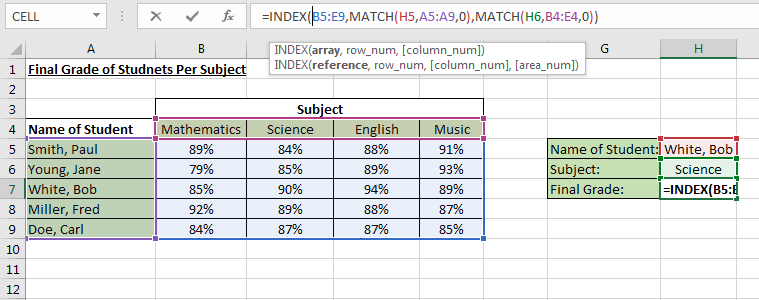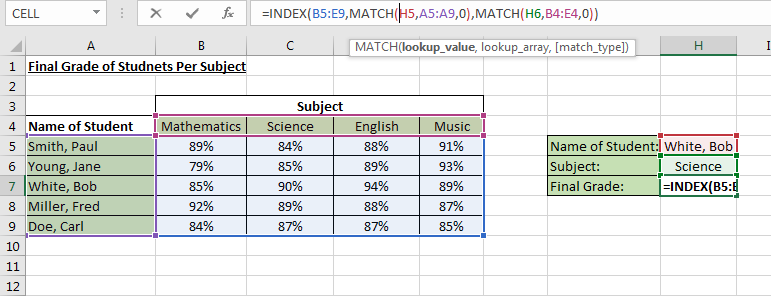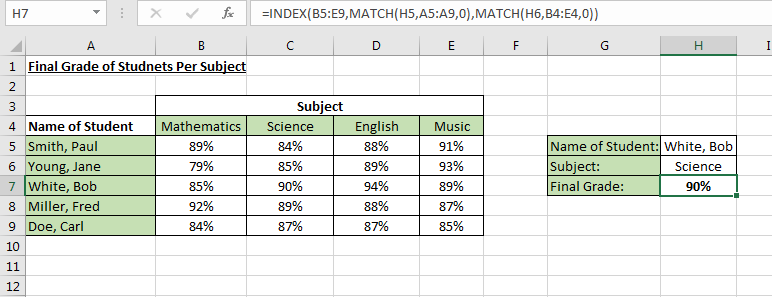# Excel Index and Match

### Index and Match Functions

This is a must know Excel tricks. The tutorial will demonstrate to find a data with two conditions out from an array. The use of Index and Match functions bridge the shortcomings of Vlookup and Hlookup wherein it can only find a value with one criteria.

Description of Example
The sets of data in the table below are the rating of each student per subject. The name of the students at column A of the table while there are corresponding ratings for each subject along row 4. The objective of this example is to find the final grade of Bob in Science.

Evaluation of Formula
Index function locates a data by mapping the position of the row and column in an array. The array is defined by ranges B5 to E9. In the figure below, it shows how the array were mapped using match functions to get the row number and column number of the array.Match function basically needs a lookup value, an array and type of match (in this case it is set to zero to find the exact match). This function were used 2 times in this example, one is to find Bob along the column of the array and the other is to find Science subject along the row of the array.Here we can see that the final grade of Bob in Science subject were pulled out from the table. Imagine how useful it is when you are working in a table with lots of data.More tutorials..
<< VBA Obfuscation | Max and If >>

Excelcrib was founded in November 2017 by Garry, a Microsoft® Office Excel® (MS Excel) enthusiast with background in engineering. He's been using MS Excel for more than 15 years in practice with specialty in VBA.